Cortix 2019 03Aug2019

# City Criminal Justice Dynamics Example¶

• This is part of the Cortix-on-Jupyter-Notebook examples.
• You must be in a Jupyter Notebook server to run this notebook.
• Select each of the cells below and run them sequentially (use the run button, >| on the tool bar or use the Cell option on the menu bar).
• Alternatively, on the menu bar run all cells: Cell -> Run All.

$\newcommand{\Amtrx}{\boldsymbol{\mathsf{A}}} \newcommand{\Bmtrx}{\boldsymbol{\mathsf{B}}} \newcommand{\Smtrx}{\boldsymbol{\mathsf{S}}} \newcommand{\xvec}{\boldsymbol{\mathsf{x}}} \newcommand{\vvar}{\boldsymbol{v}} \newcommand{\fvar}{\boldsymbol{f}} \newcommand{\Power}{\mathcal{P}} \newcommand{\bm}{{\boldsymbol{#1}}}$

## Introduction¶

This Cortix use-case simulates a population dynamics model across the network of a hypothetical criminal justice system. The mathematical model uses a population balance of different groups of individuals as described below. This work is in progress, author: Prof. Valmor F. de Almeida, UMass Lowell, Dept. of Chemical Engineering; Cortix Group.

### Prison node population model¶

The equation for the population balance in the prison node is:

\begin{equation*} d_t f_{\text{p}_g} = I_{\text{p}_g} - O_{\text{p}_g} - D_{\text{p}_g} , \end{equation*}

where $f_{\text{p}_g}(t)$ is the population for group $g$ at time $t$, $I_{\text{p}_g}(t)$ is the population inflow for group $g$, $O_{\text{p}_g}(t)$ is the outflow, and $D_{\text{p}_g}(t)$ is the death rate term in prison for a given group $g$. The inflow and outflow rates depend on the particular coupling to the prison node. For example, the contribution from the "adjudication" node (awaiting adjudication) to the prison inflow is proposed as follows:

\begin{equation*} c_\text{a,p}^{(g)} \, f_{\text{a}_g}(t) \, \mu_\text{a,p}^{(g)} , \end{equation*}

where $c_\text{a,p}^{(g)}$ is the commitment rate to "prison" from the "adjudication" for the population group $g$ (adjudication "a" to prison "p"), $f_{\text{a}_g}(t)$ is the population in the "adjudication" node, and $\mu_{\text{a}_g}$ is a conviction rate modifier. These group-dependent multiplying coefficients could be time dependent.

Hence a tentative form of inflow reads

\begin{equation*} I_{\text{p}_g}(t) = c_\text{a,p}^{(g)} \, f_{\text{a}_g}(t) \, \mu_\text{a,p}^{(g)} + c_\text{j,p}^{(g)} \, f_{\text{j}_g}(t) \, \mu_\text{j,p}^{(g)} + c_\text{e,p}^{(g)} \, f_{\text{e}_g}(t) \, \mu_\text{e,p}^{(g)} , \end{equation*}

where the last two terms refer to the "jail" node and "parole" nodes respectively. Note that the inflow is dependent on populations from distinct nodes ($f_{\text{a}_g}$, $f_{\text{j}_g}$ and $f_{\text{e}_g}$).

A tentative form for the outflow is similar but with one key difference

\begin{equation*} O_{\text{p}_g}(t) = c_\text{p,0}^{(g)} \, f_{\text{p}_g}(t) \, \mu_\text{p,0}^{(g)} + c_\text{p,e}^{(g)} \, f_{\text{p}_g}(t) \, \mu_\text{p,e}^{(g)} , \end{equation*}

that is, the rates are dependent on the population in prison. The first coefficient (or time-dependent) quantity is the unconditional rate of outflow associated to the prison population, $c_\text{p,0}^{(g)}$, and the second coefficient is its modifier $\mu_\text{p,0}^{(g)}$. The second term corresponds to the outflow of the prison population group to the parole node. This is better expressed as

\begin{equation*} O_{\text{p}_g}(t) = \bigl( c_\text{p,0}^{(g)} \, \mu_\text{p,0}^{(g)} + c_\text{p,e}^{(g)} \mu_\text{p,e}^{(g)} \big) \, f_{\text{p}_g}(t) . \end{equation*}

All together the prison population balance gives

\begin{equation*} d_t f_{\text{p}_g}(t) = \bigl( c_\text{a,p}^{(g)} \, f_{\text{a}_g}(t) \, \mu_\text{a,p}^{(g)} + c_\text{j,p}^{(g)} \, f_{\text{j}_g}(t) \, \mu_\text{j,p}^{(g)} + c_\text{e,p}^{(g)} \, f_{\text{e}_g}(t) \, \mu_\text{e,p}^{(g)} \bigr) - \bigl( c_\text{p,0}^{(g)} \, \mu_\text{p,0}^{(g)} + c_\text{p,e}^{(g)} \, \mu_\text{p,e}^{(g)} \big) \,f_{\text{p}_g}(t) - D_{\text{p}_g} , \end{equation*}

where the first term in parenthesis on the right side depends on populations on external nodes, the second term depends on the prison population, and the third term is likely a constant for each group. This population balance is non-conservative if the $D_{\text{p}_g}$ term is non-zero which is always the case; no one lives for ever, less so in prison.

### Parole node population model¶

The equation for the population balance in the parole node is similar as before:

\begin{equation*} d_t f_{\text{e}_g} = I_{\text{e}_g} - O_{\text{e}_g} - D_{\text{e}_g} , \end{equation*}

where $f_{\text{e}_g}(t)$ is the population for group $g$ at time $t$, $I_{\text{e}_g}(t)$ is the population inflow for group $g$, $O_{\text{e}_g}(t)$ is the outflow, and $D_{\text{e}_g}(t)$ is the death rate term in parole for a given group $g$. The inflow and outflow rates depend on the particular coupling to the prison node that is,

\begin{equation*} I_{\text{e}_g}(t) = c_\text{p,e}^{(g)} \, f_{\text{p}_g}(t) \, \mu_\text{p,e}^{(g)}. \end{equation*}

Note this term is the outflow term in the prison node model. Likewise the outflow term in the parole node has two contributions and it reads

\begin{equation*} O_{\text{e}_g}(t) = \bigl( c_\text{e,0}^{(g)} \, \mu_\text{e,0}^{(g)} + c_\text{e,p}^{(g)} \, \mu_\text{e,p}^{(g)} \bigr) f_{\text{e}_g}(t), \end{equation*}

the first term being the outflow to freedom and the second is a familiar term from the prison population balance. Hence overall we have

\begin{equation*} d_t f_{\text{e}_g}(t) = \bigl( c_\text{p,e}^{(g)} \, f_{\text{p}_g}(t) \, \mu_\text{p,e}^{(g)} \bigr) - \bigl( c_\text{e,0}^{(g)} \, \mu_\text{e,0}^{(g)} + c_\text{e,p}^{(g)} \, \mu_\text{e,p}^{(g)} \big) \,f_{\text{e}_g}(t) - D_{\text{e}_g} , \end{equation*}

where the first term in parenthesis on the right side depends on populations on external nodes (only the prison), the second term depends on the parole population, and the third term is likely a constant for each group. This population balance is non-conservative if the $D_{\text{e}_g}$ term is non-zero which is always the case; no one lives for ever.

The equation for the population balance in the adjudication node is similar as before also:

\begin{equation*} d_t f_{\text{a}_g} = I_{\text{a}_g} - O_{\text{a}_g} - D_{\text{a}_g} , \end{equation*}

where $f_{\text{a}_g}(t)$ is the population for group $g$ at time $t$, $I_{\text{a}_g}(t)$ is the population inflow for group $g$, $O_{\text{a}_g}(t)$ is the outflow, and $D_{\text{a}_g}(t)$ is the death rate term in adjudication for a given group $g$. The inflow and outflow rates depend on the particular coupling to the arrested ("r") node as follows:

\begin{equation*} I_{\text{a}_g}(t) = c_\text{r,a}^{(g)} \, f_{\text{r}_g}(t) \, \mu_\text{r,a}^{(g)}. \end{equation*}

Note this term will be the same as the outflow term of the arrested node model to the adjudication node model. Likewise the outflow term in the adjudication node has four contributions and it reads

\begin{equation*} O_{\text{a}_g}(t) = \bigl( c_\text{a,0}^{(g)} \, \mu_\text{a,0}^{(g)} + c_\text{a,j}^{(g)} \, \mu_\text{a,j}^{(g)} + c_\text{a,b}^{(g)} \, \mu_\text{a,b}^{(g)} + c_\text{a,p}^{(g)} \, \mu_\text{a,p}^{(g)} \bigr) f_{\text{a}_g}(t), \end{equation*}

the first term being the outflow to freedom and the other terms associated to the jail, probation, and prison nodes, respectively. Hence overall we have

\begin{equation*} d_t f_{\text{a}_g}(t) = \bigl( c_\text{r,a}^{(g)} \, f_{\text{r}_g}(t) \, \mu_\text{r,a}^{(g)} \bigr) - \bigl( c_\text{a,0}^{(g)} \, \mu_\text{a,0}^{(g)} + c_\text{a,j}^{(g)} \, \mu_\text{a,j}^{(g)} + c_\text{a,b}^{(g)} \, \mu_\text{a,b}^{(g)} + c_\text{a,p}^{(g)} \, \mu_\text{a,p}^{(g)} \big) \,f_{\text{a}_g}(t) - D_{\text{a}_g} , \end{equation*}

where the first term in parenthesis on the right side depends on a population on a external node (only the arrested node), the second term depends on the adjudication population, and the third term is likely a constant for each group. This population balance is non-conservative if the $D_{\text{a}_g}$ term is non-zero which is always the case.

### Jail node population model¶

The equation for the population balance in the jail node is similar as before also:

\begin{equation*} d_t f_{\text{j}_g} = I_{\text{j}_g} - O_{\text{j}_g} - D_{\text{j}_g} , \end{equation*}

where $f_{\text{j}_g}(t)$ is the population for group $g$ at time $t$, $I_{\text{j}_g}(t)$ is the population inflow for group $g$, $O_{\text{j}_g}(t)$ is the outflow, and $D_{\text{j}_g}(t)$ is the death rate term in jail for a given group $g$. The inflow and outflow rates depend on the particular coupling to the arrested ("r"), probation ("b") and adjudication ("a") nodes as follows:

\begin{equation*} I_{\text{j}_g}(t) = c_\text{r,j}^{(g)} \, f_{\text{r}_g}(t) \, \mu_\text{r,j}^{(g)} + c_\text{b,j}^{(g)} \, f_{\text{b}_g}(t) \, \mu_\text{b,j}^{(g)} + c_\text{a,j}^{(g)} \, f_{\text{a}_g}(t) \, \mu_\text{a,j}^{(g)} . \end{equation*}

Note this term will be the same as the outflow term of the respective models: arrested, probation and adjudication nodes model to the jail node model. Likewise the outflow term in the jail node has two contributions and it reads

\begin{equation*} O_{\text{j}_g}(t) = \bigl( c_\text{j,0}^{(g)} \, \mu_\text{j,0}^{(g)} + c_\text{j,p}^{(g)} \, \mu_\text{j,p}^{(g)} \bigr) f_{\text{j}_g}(t), \end{equation*}

the first term being the outflow to freedom and the other terms associated to the prison node, respectively. Hence overall we have

\begin{equation*} d_t f_{\text{j}_g}(t) = \bigl( c_\text{r,j}^{(g)} \, f_{\text{r}_g}(t) \, \mu_\text{r,j}^{(g)} + c_\text{b,j}^{(g)} \, f_{\text{b}_g}(t) \, \mu_\text{b,j}^{(g)} + c_\text{a,j}^{(g)} \, f_{\text{a}_g}(t) \, \mu_\text{a,j}^{(g)} \bigr) - \bigl( c_\text{j,0}^{(g)} \, \mu_\text{j,0}^{(g)} + c_\text{j,p}^{(g)} \, \mu_\text{j,p}^{(g)} \big) \,f_{\text{j}_g}(t) - D_{\text{j}_g} , \end{equation*}

where the first term in parenthesis on the right side depends on a population on a external node (only the arrested node), the second term depends on the adjudication population, and the third term is likely a constant for each group. This population balance is non-conservative if the $D_{\text{a}_g}$ term is non-zero which is always the case.

### Arrested node population model¶

The equation for the population balance in the arrested node is similar as before:

\begin{equation*} d_t f_{\text{r}_g} = I_{\text{r}_g} - O_{\text{r}_g} - D_{\text{r}_g} , \end{equation*}

where $f_{\text{r}_g}(t)$ is the population for group $g$ at time $t$, $I_{\text{r}_g}(t)$ is the population inflow for group $g$, $O_{\text{r}_g}(t)$ is the outflow, and $D_{\text{r}_g}(t)$ is the death rate term while arrested for a given group $g$. The inflow and outflow rates depend on the particular couplings to the probation, jail, adjudication, and freedom nodes that is,

\begin{equation*} I_{\text{r}_g}(t) = c_\text{0,r}^{(g)} \, f_{\text{0}_g}(t) \, \mu_\text{0,r}^{(g)}. \end{equation*}

Note this term is the outflow term in the freedom node model. Likewise the outflow term in the arrested node has four contributions and it reads

\begin{equation*} O_{\text{r}_g}(t) = \bigl( c_\text{r,0}^{(g)} \, \mu_\text{r,0}^{(g)} + c_\text{r,b}^{(g)} \, \mu_\text{r,b}^{(g)} + c_\text{r,j}^{(g)} \, \mu_\text{r,j}^{(g)} + c_\text{r,a}^{(g)} \, \mu_\text{r,a}^{(g)} \bigr) f_{\text{r}_g}(t), \end{equation*}

the first term being the outflow to freedom and the second is are by now familiar terms of the population balance. Hence overall we have

\begin{equation*} d_t f_{\text{r}_g}(t) = \bigl( c_\text{0,r}^{(g)} \, f_{\text{0}_g}(t) \, \mu_\text{0,r}^{(g)} \bigr) - \bigl( c_\text{r,0}^{(g)} \, \mu_\text{r,0}^{(g)} + c_\text{r,b}^{(g)} \, \mu_\text{r,b}^{(g)} + c_\text{r,j}^{(g)} \, \mu_\text{r,j}^{(g)} + c_\text{r,a}^{(g)} \, \mu_\text{r,a}^{(g)} \big) \,f_{\text{r}_g}(t) - D_{\text{r}_g} , \end{equation*}

where the first term in parenthesis on the right side depends on populations on external nodes (only the freedom), the second term depends on the arrested population, and the third term is likely a constant for each group. This population balance is non-conservative if the $D_{\text{r}_g}$ term is non-zero which is always the case.

### Probation node population model¶

The equation for the population balance in the probation node is similar as before:

\begin{equation*} d_t f_{\text{b}_g} = I_{\text{b}_g} - O_{\text{b}_g} - D_{\text{b}_g} , \end{equation*}

where $f_{\text{b}_g}(t)$ is the population for group $g$ at time $t$, $I_{\text{b}_g}(t)$ is the population inflow for group $g$, $O_{\text{b}_g}(t)$ is the outflow, and $D_{\text{b}_g}(t)$ is the death rate term while in probation for a given group $g$. The inflow and outflow rates depend on the particular couplings to the arrested, jail, adjudication, and freedom nodes that is,

\begin{equation*} I_{\text{b}_g}(t) = c_\text{r,b}^{(g)} \, f_{\text{r}_g}(t) \, \mu_\text{r,b}^{(g)} + c_\text{a,b}^{(g)} \, f_{\text{a}_g}(t) \, \mu_\text{a,b}^{(g)} . \end{equation*}

Note these terms are the outflow terms in the corresponding nodes model. Likewise the outflow term in the probation node has two contributions and it reads

\begin{equation*} O_{\text{b}_g}(t) = \bigl( c_\text{b,0}^{(g)} \, \mu_\text{b,0}^{(g)} + c_\text{b,j}^{(g)} \, \mu_\text{b,j}^{(g)} \bigr) f_{\text{b}_g}(t), \end{equation*}

the first term being the outflow to freedom and the second is the outflow to the jail node. Hence overall we have

\begin{equation*} d_t f_{\text{b}_g}(t) = \bigl( c_\text{r,b}^{(g)} \, f_{\text{r}_g}(t) \, \mu_\text{r,b}^{(g)} + c_\text{a,b}^{(g)} \, f_{\text{a}_g}(t) \, \mu_\text{a,b}^{(g)} \bigr) - \bigl( c_\text{b,0}^{(g)} \, \mu_\text{b,0}^{(g)} + c_\text{b,j}^{(g)} \, \mu_\text{b,j}^{(g)} \big) \,f_{\text{b}_g}(t) - D_{\text{b}_g} , \end{equation*}

where the first term in parenthesis on the right side depends on populations on external nodes (only the freedom), the second term depends on the arrested population, and the third term is likely a constant for each group. This population balance is non-conservative if the $D_{\text{r}_g}$ term is non-zero which is always the case.

### Community node population model¶

The equation for the population balance of offenders removed from the community node is similar as before:

\begin{equation*} d_t f_{\text{0}_g} = I_{\text{0}_g} - O_{\text{0}_g} - D_{\text{0}_g} , \end{equation*}

where $f_{\text{0}_g}(t) \le\ 0\ \forall\ t$ is the population for group $g$ at time $t$, $I_{\text{0}_g}(t)$ is the population inflow for group $g$, $O_{\text{0}_g}(t)$ is the outflow, and $D_{\text{0}_g}(t)$ is the death rate term while in freedom for a given group $g$. The inflow and outflow rates depend on the particular couplings to all other nodes that is,

\begin{equation*} I_{\text{0}_g}(t) = c_\text{r,0}^{(g)} \, f_{\text{r}_g}(t) \, \mu_\text{r,0}^{(g)} + c_\text{a,0}^{(g)} \, f_{\text{a}_g}(t) \, \mu_\text{a,0}^{(g)} + c_\text{p,0}^{(g)} \, f_{\text{p}_g}(t) \, \mu_\text{p,0}^{(g)} + c_\text{e,0}^{(g)} \, f_{\text{e}_g}(t) \, \mu_\text{e,0}^{(g)} + c_\text{b,0}^{(g)} \, f_{\text{b}_g}(t) \, \mu_\text{b,0}^{(g)} + c_\text{j,0}^{(g)} \, f_{\text{j}_g}(t) \, \mu_\text{j,0}^{(g)} . \end{equation*}

Note these terms are the outflow terms in the corresponding nodes model. Likewise the outflow term in the community node depends on the recidivism and creation of new offenders from the general population of non-offenders

\begin{equation*} O_{\text{0}_g}(t) = c_\text{0,r}^{(g)} \, \mu_\text{0,r}^{(g)} \, \left|f_{\text{0}_g}(t)\right| + c_\text{0,0}^{(g)} \, \mu_\text{0,0}^{(g)} \, f_{\text{0}}(t) \end{equation*}

the outflow of the community only takes place to an arrested node. It depends on the population of removed offenders and the non-offenders population where $f_{\text{0}}(t)$ is a given function from a population dynamics model in the entire community; it couples with other urban dynamic models if any. $f_{\text{0}}(t)$ could be taken as a constant number of individuals reaching maturity age. Hence overall we have

\begin{equation*} d_t f_{\text{0}_g}(t) = \bigl( c_\text{r,0}^{(g)} \, f_{\text{r}_g}(t) \, \mu_\text{r,0}^{(g)} + c_\text{a,0}^{(g)} \, f_{\text{a}_g}(t) \, \mu_\text{a,0}^{(g)} + c_\text{p,0}^{(g)} \, f_{\text{p}_g}(t) \, \mu_\text{p,0}^{(g)} + c_\text{e,0}^{(g)} \, f_{\text{e}_g}(t) \, \mu_\text{e,0}^{(g)} + c_\text{b,0}^{(g)} \, f_{\text{b}_g}(t) \, \mu_\text{b,0}^{(g)} + c_\text{j,0}^{(g)} \, f_{\text{j}_g}(t) \, \mu_\text{j,0}^{(g)} \bigr) - \bigl( c_\text{0,r}^{(g)} \, \mu_\text{0,r}^{(g)} \,\left|f_{\text{0}_g}(t)\right| + c_\text{0,0}^{(g)} \, \mu_\text{0,0}^{(g)} \, f_{\text{0}}(t) \bigr) - D_{\text{b}_g} , \end{equation*}

where the first term in parenthesis on the right side depends on populations on external nodes, the second term depends on the offending population in the community, and the next term depends on the population reaching the maturity age. The last term is likely a constant for each group. This population balance is non-conservative if the $D_{\text{0}_g}$ term is non-zero which is always the case.

## Write the run context¶

In :
# Import various packages; must have the Cortix repository installed

import matplotlib.pyplot as plt
import scipy.constants as const

# Leave this block here for Azure
try:
import cortix
except ImportError:
print('Installing the "cortix" package...')
print('')
!pip install cortix
import cortix

from cortix.src.cortix_main import Cortix
from cortix.src.network import Network

from cortix.examples.city_justice.prison import Prison
from cortix.examples.city_justice.parole import Parole
from cortix.examples.city_justice.jail import Jail
from cortix.examples.city_justice.arrested import Arrested
from cortix.examples.city_justice.probation import Probation
from cortix.examples.city_justice.community import Community

In :
# Setup parameters
end_time  = 50 * const.day
time_step = 0.5 * const.day
n_groups  = 450   # number of population groups; these are typically large
# cartesian product of individual attributes, say:
#    age (in groups, say 5 groups),
#    gender,
#    race/etnicity,
#    number of prior felony convictions,
#    violence in prior felony,
#    sex offence in prior felony,
#    current conviction offense
# e.g. 18-24 year old, white male, one prior, violent prior, drug offense, sex offense, etc.

# Create a Cortix object with Python multiprocessing
city = Cortix(use_mpi=False,splash=True)

city.network = Network()
city_net = city.network

 2019-10-23 14:42:49,868 - cortix - INFO - Created Cortix object
_____________________________________________________________________________
L A U N C H I N G
_____________________________________________________________________________
...                                        s       .     (TAAG Fraktur)
xH88"~ .x8X                                 :8      @88>
:8888   .f"8888Hf        u.      .u    .      .88      %8P      uL   ..
:8888>  X8L  ^""   ...ue888b   .d88B :@8c    :888ooo    .     [email protected]  @88R
X8888  X888h        888R Y888r ="8888f8888r -*8888888  [email protected]  ""Y888k/"*P
88888  !88888.      888R I888>   4888>"88"    8888    888E    Y888L
88888   %88888      888R I888>   4888> "      8888      888E      8888
88888 > 8888>     888R I888>   4888>        8888      888E      888N
8888L %  ?888   ! u8888cJ888   .d888L .+    .8888Lu=   888E   .u./"888&
8888  -*""   /   "*888*P"    ^"8888*"     ^%888*     888&  d888" Y888*"
"888.      :"      "Y"          "Y"         "Y"      R888"  "Y   Y"
""***~"                                           ""
https://cortix.org
_____________________________________________________________________________

In :
# Create the application network

city_net.module(community)
community.end_time = end_time
community.time_step = time_step
community.show_time = (True,10*const.day)
community.save = True

prison = Prison(n_groups=n_groups, pool_size=0)
city_net.module(prison)
prison.end_time = end_time
prison.time_step = time_step
prison.save = True

parole = Parole(n_groups=n_groups, pool_size=0)
city_net.module(parole)
parole.end_time = end_time
parole.time_step = time_step
parole.save = True

jail = Jail(n_groups=n_groups, pool_size=0)
city_net.module(jail)
jail.end_time = end_time
jail.time_step = time_step
jail.save = True

arrested = Arrested(n_groups=n_groups, pool_size=0)
city_net.module(arrested)
arrested.end_time = end_time
arrested.time_step = time_step
arrested.save = True

probation = Probation(n_groups=n_groups, pool_size=0)
city_net.module(probation)
probation.end_time = end_time
probation.time_step = time_step
probation.save = True

city_net.connect( prison, parole,'bidirectional' )
city_net.connect( jail, prison )
city_net.connect( arrested, jail )
city_net.connect( arrested, probation )
city_net.connect( probation, jail )
city.save = True

city_net.connect( prison, community )
city_net.connect( jail, community )
city_net.connect( arrested, community, 'bidirectional' )
city_net.connect( parole, community )
city_net.connect( probation, community )


## Verify the network connectivity¶

In :
# View the Cortix network created

city_net.draw()

Out:

## Run network simulation¶

In :
# Run the simulation!

city.run()

 2019-10-23 14:42:50,158 - cortix - INFO - Launching Module <cortix.examples.city_justice.community.Community object at 0x1514923710>
 2019-10-23 14:42:50,165 - cortix - INFO - Launching Module <cortix.examples.city_justice.prison.Prison object at 0xf146bbeb8>
 2019-10-23 14:42:50,168 - cortix - INFO - Community::time[d] = 0.0
 2019-10-23 14:42:50,172 - cortix - INFO - Launching Module <cortix.examples.city_justice.parole.Parole object at 0x1514923780>
 2019-10-23 14:42:50,182 - cortix - INFO - Launching Module <cortix.examples.city_justice.adjudication.Adjudication object at 0x15149236d8>
 2019-10-23 14:42:50,189 - cortix - INFO - Launching Module <cortix.examples.city_justice.jail.Jail object at 0x1514923748>
 2019-10-23 14:42:50,201 - cortix - INFO - Launching Module <cortix.examples.city_justice.arrested.Arrested object at 0x15148fce80>
 2019-10-23 14:42:50,212 - cortix - INFO - Launching Module <cortix.examples.city_justice.probation.Probation object at 0x1514923d68>
 2019-10-23 14:42:50,638 - cortix - INFO - Community::time[d] = 10.0
 2019-10-23 14:42:50,925 - cortix - INFO - Community::time[d] = 20.0
 2019-10-23 14:42:51,299 - cortix - INFO - Community::time[d] = 30.0
 2019-10-23 14:42:51,660 - cortix - INFO - Community::time[d] = 40.0
 2019-10-23 14:42:52,186 - cortix - INFO - run()::Elapsed wall clock time [s]: 2.32


## Results inspection through Cortix¶

In :
community     = city_net.modules
prison             = city_net.modules
parole             = city_net.modules
jail                   = city_net.modules
arrested          = city_net.modules
probation        = city_net.modules
'Jail':'fjg','Arrested':'frg','Probation':'fbg','Community':'f0g'}

total_num_params = 0

In :
'''Inspect Data Function'''
def inspect_module_data(module,quant_names):
population_phase = module.population_phase
if isinstance(quant_names,dict):
(fxg_quant, time_unit) = population_phase.get_quantity_history(quant_names[module.name])
elif isinstance(quant_names,str):
(fxg_quant, time_unit) = population_phase.get_quantity_history(quant_names)
fxg_quant.plot( x_scaling=1/const.day, x_label='Time [day]', y_label=fxg_quant.name+' ['+fxg_quant.unit+' %]')
plt.grid()
plt.show()

# number of parameters in the prison model
n_params = (len(population_phase.GetActors())-1)*n_groups
print('# parameters = ',n_params)
return n_params


### Prison module¶

In :
total_num_params += inspect_module_data(prison,quant_names)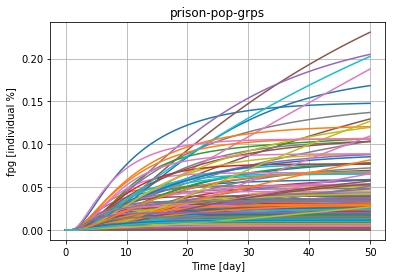# parameters =  1800


### Parole module¶

In :
total_num_params += inspect_module_data(parole,quant_names)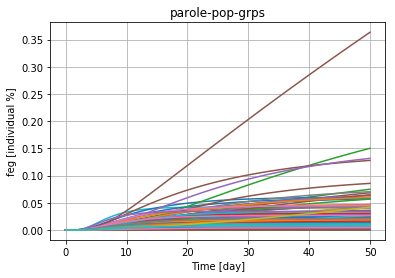# parameters =  1800


In :
total_num_params += inspect_module_data(adjudication,quant_names)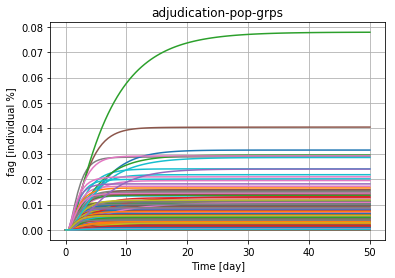# parameters =  3600


### Jail module¶

In :
total_num_params += inspect_module_data(jail,quant_names)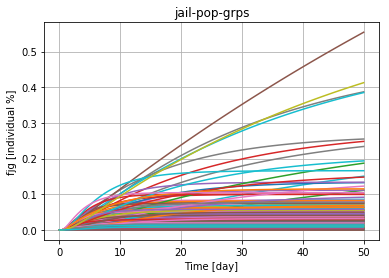# parameters =  1800


### Arrested module¶

In :
total_num_params += inspect_module_data(arrested,quant_names)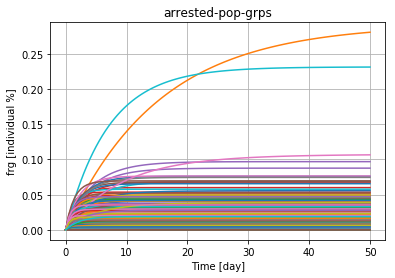# parameters =  3600


### Probation module¶

In :
total_num_params += inspect_module_data(probation,quant_names)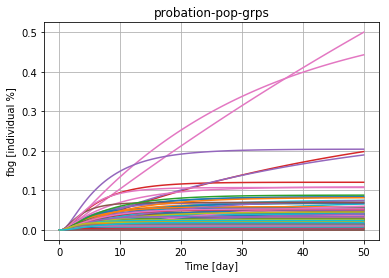# parameters =  1800


### Community module¶

Offenders removed (negative sign) from the general non-offender community.

In :
total_num_params += inspect_module_data(community,quant_names)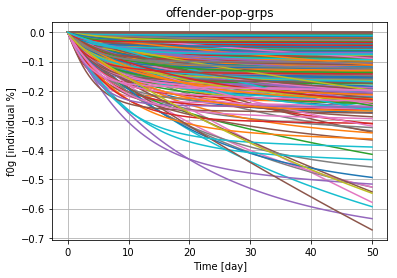# parameters =  2250

In :
total_num_params += inspect_module_data(community,'f0g_free')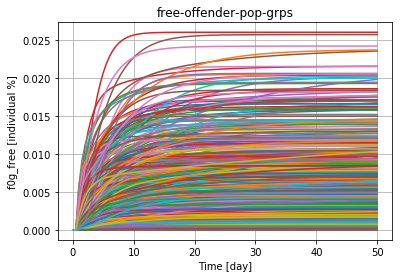# parameters =  2250

In :
'''Total number of unknowns and parameters'''

print('total number of unknowns   =', n_groups*len(city_net.modules))
print('total number of parameters =', total_num_params)

total number of unknowns   = 3150
total number of parameters = 18900